Test: Boundary Layer Theory - 3

# Test: Boundary Layer Theory - 3

Test Description

## 10 Questions MCQ Test Fluid Mechanics | Test: Boundary Layer Theory - 3

Test: Boundary Layer Theory - 3 for Mechanical Engineering 2022 is part of Fluid Mechanics preparation. The Test: Boundary Layer Theory - 3 questions and answers have been prepared according to the Mechanical Engineering exam syllabus.The Test: Boundary Layer Theory - 3 MCQs are made for Mechanical Engineering 2022 Exam. Find important definitions, questions, notes, meanings, examples, exercises, MCQs and online tests for Test: Boundary Layer Theory - 3 below.
Solutions of Test: Boundary Layer Theory - 3 questions in English are available as part of our Fluid Mechanics for Mechanical Engineering & Test: Boundary Layer Theory - 3 solutions in Hindi for Fluid Mechanics course. Download more important topics, notes, lectures and mock test series for Mechanical Engineering Exam by signing up for free. Attempt Test: Boundary Layer Theory - 3 | 10 questions in 30 minutes | Mock test for Mechanical Engineering preparation | Free important questions MCQ to study Fluid Mechanics for Mechanical Engineering Exam | Download free PDF with solutions
 1 Crore+ students have signed up on EduRev. Have you?
Test: Boundary Layer Theory - 3 - Question 1

### The hydrodynamic boundary layer thickness is defined as the distance from the surface where the

Detailed Solution for Test: Boundary Layer Theory - 3 - Question 1

Hydrodynamic boundary layer thickness is defined as the distance measured in the y direction from boundary to the point where the velocity is 99% of the free stream velocity.

Test: Boundary Layer Theory - 3 - Question 2

### Laminar boundary layer thickness (δ) at any point x for flow over a flat plate is given by δ/x

Detailed Solution for Test: Boundary Layer Theory - 3 - Question 2

From the Blasius solution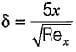Test: Boundary Layer Theory - 3 - Question 3

### The velocity profile for turbulent layer over a flat plate is

Detailed Solution for Test: Boundary Layer Theory - 3 - Question 3

Turbulent velocity profile follows 1/7 power law, hence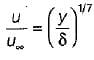will be velocity distribution.

Test: Boundary Layer Theory - 3 - Question 4

The turbulent boundary layer thickness varies as

Detailed Solution for Test: Boundary Layer Theory - 3 - Question 4

For turbulent flow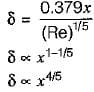Test: Boundary Layer Theory - 3 - Question 5

According to Blasius, the local skin friction coefficient in the boundary layer over a flat plate is given by

Detailed Solution for Test: Boundary Layer Theory - 3 - Question 5

Local skin friction coefficient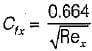Test: Boundary Layer Theory - 3 - Question 6

The thickness of laminar boundary layer at a distance 'x' from the leading edge over a flat plate varies as

Detailed Solution for Test: Boundary Layer Theory - 3 - Question 6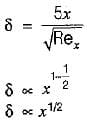Test: Boundary Layer Theory - 3 - Question 7

The Von-karman momentum integral equation expressed as (where θ is momentum thickness)

Detailed Solution for Test: Boundary Layer Theory - 3 - Question 7

Von-karman momentum integral equation is expressed as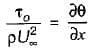Test: Boundary Layer Theory - 3 - Question 8

Consider the following statements:
Boundary layer separation is caused by:
2. Sudden entrapping of air
3. Reduction of pressure gradient to zero
4. Release of bubbles from the fluid when the pressure goes below the vapor pressure

Q. Which of the above statements is/are correct?

Test: Boundary Layer Theory - 3 - Question 9

Air flows past a golf bail of 20 mm radius. It is observed that the boundary layer becomes turbulent at Reynolds number of 2 x 105. If the kinematic viscosity of air is 1.5 x 10-5 m2/sec then the speed of air when the flow becomes turbulent is

Detailed Solution for Test: Boundary Layer Theory - 3 - Question 9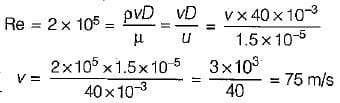Test: Boundary Layer Theory - 3 - Question 10

Blasius equation for a flat plate laminar boundary layer flow is a third order​

## Fluid Mechanics

50 videos|89 docs|76 tests
 Use Code STAYHOME200 and get INR 200 additional OFF Use Coupon Code
Information about Test: Boundary Layer Theory - 3 Page
In this test you can find the Exam questions for Test: Boundary Layer Theory - 3 solved & explained in the simplest way possible. Besides giving Questions and answers for Test: Boundary Layer Theory - 3, EduRev gives you an ample number of Online tests for practice

## Fluid Mechanics

50 videos|89 docs|76 tests